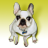# Big O cost of computing drive shed/service area

616
5
03-01-2012 10:42 AMNew Contributor
Can someone tell me what is the cost of calculating the drive shed/service area in network analyst (big 0 notation)?  Is this an NP problem? NP Complete?
Tags (2)
1 Solution

Accepted SolutionsNew Contributor III
The "service area" problem is merely a single-source shortest path search with cutoffs (i.e., starting from each facility [the "source"], find the parts of the network that can be reached within travel cost <= x units, for some user-specified cutoff value of x). Therefore, it can be solved in polynomial time using straightforward variants of Dijkstra's algorithm (assuming non-negative edge weights), which, for graphs with n nodes and m edges, runs in O(mlogn) time if using binary heaps for the priority queue data structure or in O(m + nlogn) time if using Fibonacci heaps for the priority queue.
5 RepliesNew Contributor III
The "service area" problem is merely a single-source shortest path search with cutoffs (i.e., starting from each facility [the "source"], find the parts of the network that can be reached within travel cost <= x units, for some user-specified cutoff value of x). Therefore, it can be solved in polynomial time using straightforward variants of Dijkstra's algorithm (assuming non-negative edge weights), which, for graphs with n nodes and m edges, runs in O(mlogn) time if using binary heaps for the priority queue data structure or in O(m + nlogn) time if using Fibonacci heaps for the priority queue.New Contributor
Isnt that algorithm for the distance between two points, where as a service area is going out in every direction on every node until 'time runs out' so to speak?New Contributor III
Dijkstra's algorithm works for both of the cases you point out. The generic version of Dijkstra's algorithm searches to find the shortest path from a source node to all other nodes in the graph. Therefore, it does actually "go out in every direction" as you suggest.

However, if we are only looking for the shortest path to a specific node, the algorithm can simply terminate as soon as it finds the shortest path to that particular node (instead of continuing the search for all remaining nodes).

For service area, the search goes out in all directions until it has processed all nodes within the specified cutoff, at which point it terminates (note that this termination criterion is easy to detect because Dijkstra's algorithm processes nodes in monotonically increasing order of their shortest path cost from the source). The nodes within the cutoff range and the edges taken to reach them define the service area (which can be output as lines or as polygons covering these lines).New Contributor
thanks for the clarification 😃New Contributor III